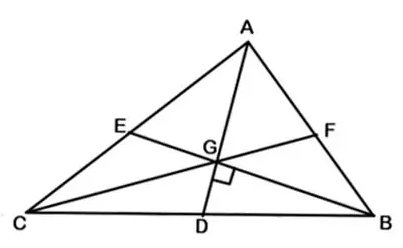# In a triangle ABC, medians AD | Quantitative Aptitude – Geometry

## CAT 2019 – Slot -2 - Question 1 - In a triangle ABC, medians AD

Q. 1: In a triangle ABC, medians AD and BE are perpendicular to each other, and have lengths 12 cm and 9 cm, respectively. Then, the area of triangle ABC, in sq cm, is
1. 80
2. 68
3. 72
4. 78Draw the third median CF. As We know that the :

1. The intersection point of medians i.e. centroid (G) divides each median into 2:1.

2. All three medians divide the triangle into 6 triangle having equal area.

So Area of Triangle ABC = 6* Area of one small triangle—————-1)

Now Given AD = 12 and GD:AG = 1:2 So GD = 1/3 *12 =4

BE = 9 and BG:GE = 2:1 So BG = 2/3 *9 = 6

As BG is perpendicular to GD,

Area of triangle BGD = ½ ×GB × GD = ½ × 6 × 4 =12

Hence, from eq 1) area of triangle ABC = 6 × 12 = 72

### Past Year Question Paper & SolutionsCounselling Session
By IIM Mentor

#### Free Material Area